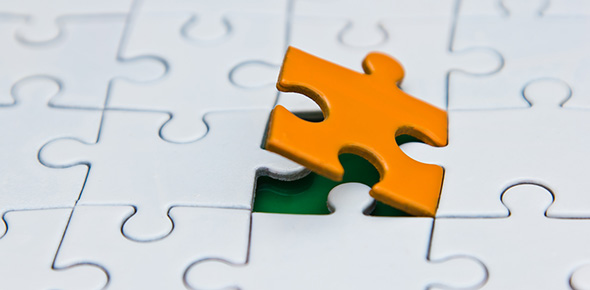# Exit Exam Gcsu Spring 2015

75 Questions | Total Attempts: 53Settings.

• 1.
What is the average rest RER value for adults?
• A.

.80-.85

• B.

.78-.80

• C.

.70-.75

• D.

.60-.70

• 2.
The combustion of fat requires less oxygen than carbohydrates?
• A.

True

• B.

False

• 3.
The ratio between the amount of CO2 released and the O2 consumed in one breath?
• 4.
The volume of air expired per minute?
• A.

Minute volume

• B.

Minute ventilation

• C.

Pulmonary ventilation

• D.

VO2

• 5.
Training causes RER to __________ and results in more utilization of FFA during submaximal exercise.
• 6.
__________ is measured as the amount of blood ejected from the left ventricle during contraction.
• 7.
Highly trained athletes can reach a ejection fraction of close to 100%?
• A.

True

• B.

False

• 8.
________ is the proportion of blood within the heart compared to that which gets pumped out.
• 9.
What are the two measurements of Work we are concerned with in exercise science?
• A.

VO2 (mL/kg/min

• B.

Watts

• C.

METS

• D.

Mass (kg)

• 10.
Two athletes working out have the same cardiac output, but athlete A has a lower heart rate than athlete B. Which athlete will last longer during a competition?
• A.

Athlete A

• B.

Athlete B

• C.

They will last the same amount of time

• D.

Not enough information is present to answer the question

• 11.
At what percent of VO2 max does Stroke Volume plateau during exercise?
• A.

35%

• B.

50%

• C.

75%

• D.

95%

• 12.
Training increases plasma volume in blood.
• A.

True

• B.

False

• 13.
The blood contents of Hematocrit are also known as ______.
• 14.
Exercise technique described by closing the glottis, mouth, and nose during exhalation to increase intrathoracic pressure.
• 15.
The highest SV attainable in the upright position is much greater than the resting value in the supine position.
• A.

True

• B.

False

• 16.
This measures the amount of oxygen extracted by the tissues from blood during one trip around the systemic circulation.
• 17.
Healthy adults eject what volume of oxygen per dL of blood from the heart?
• A.

15 mL

• B.

30 mL

• C.

20 mL

• D.

5 mL

• 18.
What percentage of oxygen saturation in hemoglobin is considered optimal?
• A.

98-100%

• B.

89-90%

• C.

93-95%

• D.

75-80%

• 19.
The Cardiac Output at rest is between 5-6L of blood at rest.
• A.

True

• B.

False

• 20.
Increases in exercise intensity are associated with a(n) __________ in (a-v)O2 difference
• 21.
What equation is used to determine the rate at which oxygen is being used during activity?
• A.

Ventilatory Threshold Equation

• B.

Karvonen Equation

• C.

Systemic Equation

• D.

Fick Equation

• 22.
Cardiac Output can reach volumes of 18 -25 L/min at max exercise?
• A.

True

• B.

False

• 23.
What is the total amount of blood pumped through the left ventricle per minute?
• 24.
Is it possible to lose type IIX muscle fibers?
• A.

Yes, they are converted as we age

• B.

No, muscle fibers cannot change types

• 25.
Select the following functions of the cardiovascular system?
• A.

Delivery of Nutrients

• B.

Removal of Wastes

• C.

Transport of Oxygen and Hormones

• D.

Maintain Acid /Base Concentrations

• E.

Maintain Homeostasis

• F.

Pump Blood throughout the body

• G.

Produce work by the body

• H.

Signal impulses to produce movement actionsBack to top